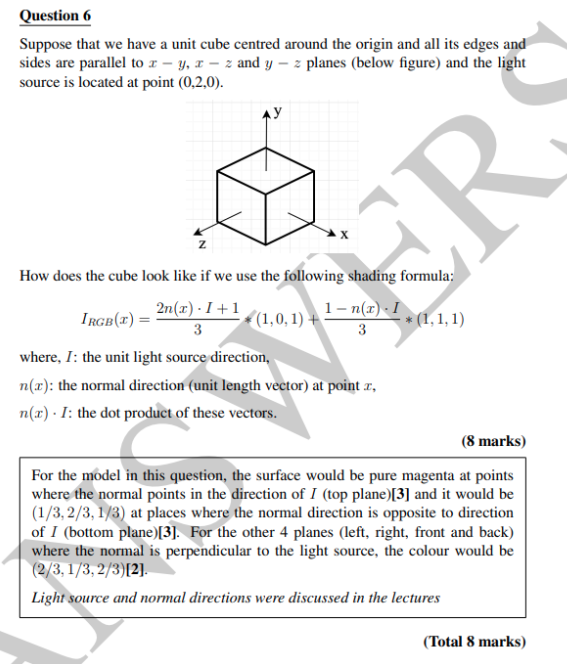# With a light source at (0,2,0) and unit cube (from [-1,1]) using a given shading formula, how is the r,g,b for each surface calculated?The above problem is from a past Computer Graphics exam.

I am particularly confused about the explanation given, firstly for the top and bottom planes (since the direction of normal for top plane seems to be opposite the direction of I)

I tried to calculate the normal vectors, for the top plane for instance, by choosing two arbitrary points on the top plane ([1,1,1] and [0,1,0]), subtracting the two point vectors to get the vector lying across the plane. I then used the fact that the normal vector dot product with the vector lying across the plane equals zero to get a linear equation of the plane in the form Ax + By + Cz = 0, and used that to try to find the normal vector from the A,B,C components to multiply by the unit vector to yield the normal vector. This seems to be incorrect to me since it gave a normal vector defined as (1,0,1), which intuitively seems incorrect.

I do intuitively understand that the normal vector from the top plane will be positive in the y axis, and the normal vector from the bottom plane will be negative in the y axis. I can also see that a vector in the positive y axis would go towards the magenta light source so the plane that defines that normal vector would be illuminated. I do not understand how the specific values were computed.

If possible, an explanation of how they obtained values for the top plane, bottom plane, and 4 other planes would be appreciated. Thanks.

• Why would you calculate normal vectors? The question tells you that the cube is aligned with the axes. Which means the normals are the standard unit vectors. For top plane, this is simply $(0,1,0)$. The Light source is at $(0,2,0)$ If you take the unit vector for this it's the same as $(0, 1, 0)$. Just plug this in the formula and you'll get the color for the plane. Repeat the steps for other planes. May 6 at 3:28
• Thank you, that makes sense. May 7 at 13:35

First of all, it is obvious that the normal vector of the upper surface is $$(0,1,0)$$. But you can calculate it in the following way:

Given three points on a surface that are not aligned, you can calculate the normal direction. A very simple way is to use the cross product $$n = |(p1 - p0)$$ x $$(p2 - p0)|$$ . With this formula you have to be careful which points you choose for $$p0$$, $$p1$$ and $$p2$$, because the cross product gives the orthogonal direction of the two input vectors according to the right-hand rule.

What is the right hand rule?Figure 1: Copied from Wikipedia. Shows how to easily remember the direction of the result of a cross product. (according to the right hand rule (Do NOT use the left hand ;P ))

So the result of $$a$$ x $$b$$ is not the same as $$b$$ x $$a$$. So the cross product is not commutative! When calculating the normal vector, this should be taken into account! The length of the result of the cross product must be normalized.

Once we have the normal vectors of all 6 surfaces, we can proceed with the formula. $$I_{RGB}(x) = \frac{2n(x) * I + 1}{3} * (1,0,1) + \frac{1-n(x)*I}{3} * (1,1,1)$$ The question of the professor is not well defined, because the light source direction vary depending on the position of the point on surface... I would expect a well divined question from a university... The question could be defined when saying: the light source is infinity far away, which leeds to a directional light source. Or in case inside the course you only talked about directional light sources, they need to define the light cast direction. But when looking at the answer of the professor we see, that he/she expect you to use the direction of: $$|lightPosition - centerCube|$$. Therefore we know the value of $$I = (0,1,0)$$ (where, $$I$$: the unit light source direction,) (so this vectors is normalized). So if we substitute $$I$$ into the equation, we get: $$I_{RGB}(x) = \frac{2n(x) * (0,1,0) + 1}{3} * (1,0,1) + \frac{1-n(x)*(0,1,0)}{3} * (1,1,1)$$

Lets start with the top face: $$n = (0,1,0)$$ So placing the vector into the formular: $$I_{RGB}(x) = \frac{2(0,1,0) * (0,1,0) + 1}{3} * (1,0,1) + \frac{1-(0,1,0)*(0,1,0)}{3} * (1,1,1)$$ because $$n(x)*I$$ is the dot product, we can shorten the equation: $$I_{RGB}(x) = \frac{2 + 1}{3} * (1,0,1) + \frac{1-1}{3} * (1,1,1)$$ $$= 1 * (1,0,1) + 0 * (1,1,1)$$ $$= (1,0,1)$$ $$(1,0,1)$$ as RGB value is pure magenta.

Lets continue with the bottom face: $$n = (0,-1,0)$$ So placing the vector into the formular: $$I_{RGB}(x) = \frac{2(0,-1,0) * (0,1,0) + 1}{3} * (1,0,1) + \frac{1-(0,-1,0)*(0,1,0)}{3}*(1,1,1)$$ Lets shorten it like above, gives you: $$I_{RGB}(x) = \frac{-2 + 1}{3} * (1,0,1) + \frac{1-(-1)}{3}*(1,1,1)$$ $$= (-\frac{1}{3},0,-\frac{1}{3}) + (\frac{2}{3},\frac{2}{3},\frac{2}{3})$$ $$= (\frac{1}{3},\frac{2}{3},\frac{1}{3})$$

Lets continue with the front face: $$n = (0,0,1)$$ So placing the vector into the formular: $$I_{RGB}(x) = \frac{2(0,0,1) * (0,1,0) + 1}{3} * (1,0,1) + \frac{1-(0,0,1)*(0,1,0)}{3}*(1,1,1)$$ Lets shorten it like above, gives you: $$I_{RGB}(x) = \frac{0 + 1}{3} * (1,0,1) + \frac{1-0}{3}*(1,1,1)$$ $$= (\frac{1}{3},0,\frac{1}{3}) + (\frac{1}{3},\frac{1}{3},\frac{1}{3})$$ $$= (\frac{2}{3},\frac{1}{3},\frac{2}{3})$$

I'll stop here, for the other 3 faces it works the same way...

• Excellent explanation, thank you! May 7 at 13:34
• You are welcome :) May 7 at 16:07